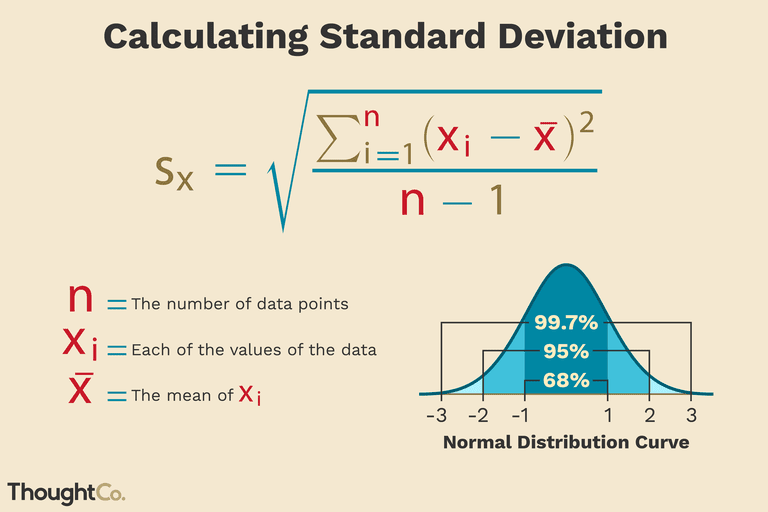The world's leading A.I. powered trading network• home
•  Blog
• The Deviance of the Standard Deviation

# The Deviance of the Standard Deviation

Square Root RuleDon’t panic I am going to try and keep this simple.

Howard Wainer is quoted as saying that the formula for the standard deviation of the mean is the “most dangerous equation in the world” allow me to show you why. I need to pay tribute to Professor Scott Page and his book The Model Thinker from where I am drawing this insight.

I am going to skip how this fits in with the Central Limit Theorem and the Square Root Rules and dive straight into showing how grasping the little bit I am going to share in this letter will help you view the world of statistics in a more informed light than many so called experts.

When dealing with normal distributions exceptional outcomes occur far more frequently when dealing with small sample sizes. The reason is staring us in the formula’s face: The formula for the standard deviation of the mean implies that large populations have much lower standard deviations than small ones.

Let us look at a real life example.

In the 1990’s the Gates Foundation advocated breaking up schools into smaller schools based on evidence that the best schools were small. Even the brilliant Gates Foundation screwed this one up and had to finally revise their findings.

Imagine that schools come in two sizes 100 (small) students and 1600 (large) students. Student scores from both schools have a mean of 100 and a standard deviation of 80.

Small Schools

The standard deviation of the mean = 8 ( std deviation, 80 / square root of students, 10).

Large Schools

The standard deviation of the mean = 2 ( std deviation, 80 / square root of students, 40).

If we classify a school as “Exceptional” with a mean of 124 let us do some math:

Small Schools

124 – 100 = 24 / 8 = 3 standard deviations above the mean = to a probability of 1 school in 370 displays exceptional characteristics.

Large Schools

124 – 100 = 24 / 2 = 12 standard deviations above the mean = to a probability of 1 school in so many billions it is impossible to count.

The mistaken takeaway from this statistical analysis is that you will think that Exceptional Schools have to be Small Schools.

Mike’s 2 Takeaways

I am sure there are many more, let me start with a reference to the PsyQuation Score™.

1. Some people have questioned/criticized our score because it seems to favour traders that trade a lot versus traders that trade very infrequently. Our Score is designed with a statistical philosophy in mind and it very mindful of the dangers of incorrectly classifying a small sample distribution of returns. This is why our Score grows in confidence with the more trades done. [don’t panic, above a certain threshold say 300 trades, there is a diminishing marginal utility function, meaning the contribution of more trades contributes less and less to the overall score]
2. Be very very careful drawing too much from a single backtest or a trade setup with a small sample size of historic similarities. The small sample size is likely to overstate the likelihood of these events happening in the future.